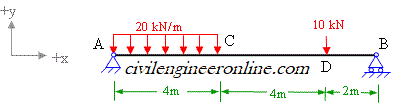Slope and deflection Calculation by Macaulay's method

Problem 6-2

Use Macaulay's method to determine the values of slope and deflection at 5m from the left support due to imposed load as shown in figure 6-2(a). The material of beam has modulus of elasticity as 200 GPa. The beam cross-section is I-shaped with top and bottom flange width as 250 mm and thickness 20 mm, web height as 300 mm and web thickness as 15 mm.Figure 6-2(a)

Solution:

The loading is not same throughout the span, therefore we use Macaulay's Method for finding slope and deflection of the given beam.

The given beam has two unknown reaction components which are calculated in Prob 4-1 as  Ay = 66 kN and By = 24 kN;Figure 6-2(b)

The differential equation of elastic curve for this  beam can be written as follows;

EI (d2y/dx2) = 66[x] - 20[x]2 /2 +20[x-4]2/2 - 10[x-8]         Eq. 1

Integrating Eq. 1 we get;

EI(dy/dx) = 66[x]2/2 - 20[x]3 /6 +20[x-4]3/6 - 10[x-8]2/2 +C1     Eq.2

We do not have any information about slope at the ends,

We continue integrating Eq. 2 for finding deflection;

EIy = 66[x]3/6 - 20[x]4 /24 +20[x-4]4/24 - 10[x-8]3/6 +C1x + C2

Now apply the condition for deflection at the supports;

at x=0, y=0 (neglect the terms which become negative with x=0)

we get C2=0;

Further at x=10, y=0; we get

0=11000 - 200000/24 + 1080 - 80/6 + 10C1

C1= -374.66

Therefore the equation for slope can be written as

EI(dy/dx) = 66[x]2/2 - 20[x]3 /6 +20[x-4]3/6 - 10[x-8]2/2 - 374.66

and the equation for deflection would be

EIy = 66[x]3/6 - 20[x]4 /24 +20[x-4]4/24 - 10[x-8]3/6 - 374.66x

Slope at x=5;

dy/dx = (37)/EI

Deflection at x=5;

y = (- 1018)/EI

The value of moment of inertia can be calculated by using moment of inertia calculator

Ixx = 29008.3 cm4

EI= (200x109 N/m2)x(29008.3 cm4 ) = 58016.6 kN m2

substituting the value of EI in the expressions for slope and deflection we get;

dy/dx = 37/58016.6 = 0.00064 rad.

y = - 1018/58016.6 = - 0.0175 m (negative sign indicates that the deflection is downward)

You can also use our Slope deflection calculator for different combinations of load on Simple beam.

#### Excellent Calculators

Stress Transformation Calculator
Calculate Principal Stress, Maximum shear stress and the their planes

To determine Absolute Max. B.M. due to moving loads.

Bending Moment Calculator
Calculate bending moment & shear force for simply supported beam

Moment of Inertia Calculator
Calculate moment of inertia of plane sections e.g. channel, angle, tee etc.

Reinforced Concrete Calculator
Calculate the strength of Reinforced concrete beam

Moment Distribution Calculator
Solving indeterminate beams

Deflection & Slope Calculator
Calculate deflection and slope of simply supported beam for many load cases

Fixed Beam Calculator
Calculation tool for beanding moment and shear force for Fixed Beam for many load cases

BM & SF Calculator for Cantilever
Calculate SF & BM for Cantilever

Deflection & Slope Calculator for Cantilever
For many load cases of Cantilever

Overhanging beam calculator
For SF & BM of many load cases of overhanging beam

Civil Engineering Quiz
Test your knowledge on different topics of Civil Engineering

Research Papers
Research Papers, Thesis and Dissertation

List of skyscrapers of the world
Containing Tall buildings worldwide

Forthcoming conferences
Containing List of civil engineering conferences, seminar and workshops

Profile of Civil Engineers
Get to know about distinguished Civil Engineers

Professional Societies
Worldwide Civil Engineers Professional Societies